## [原创]最小二乘的理论依据

在做数据建模或者曲线拟合的时候，我们通常会用到最小二乘法。假设作为数学模型的函数为 $y = f(x,S)$ ，其中 $S$ 为参数集向量（即一系列的参数）， $x$ 为自变量。在这种情况下，为了求出 $S$ ，需要对下式进行极小化：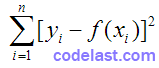即：对已知的一个数据集 ${x_i}(i = 1,2, \cdots ,n)$ ，能极小化该式的 $S$ 就是最优参数。但是这个式子是怎么来的呢？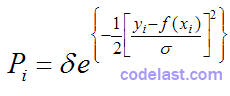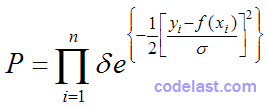如前文所述：对参数 $S$ ，能使已知数据集发生的概率越大，那么就说明我们取的 $S$ 越优良。因此，使上式最大化就是我们的目标。由于 $\delta$ 为正常数， $f(x) = {e^x}$ 为单调递增函数，因此，想要：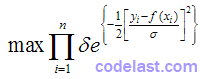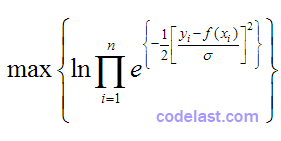等同于：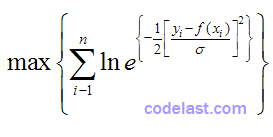继续化简：相当于：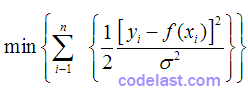现在，由于 $\sigma$ 是常数，上式就等同于：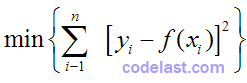这就得到了我们要推导的结论。

NULL